﻿ Digital Circuits and Systems - Circuits i Sistemes Digitals (CSD) - EETAC - UPCBachelor's Degree in Telecommunications Systems and in Network Engineering## P5: Analysis of circuits based on flip-flops, latches and logic gates

 Resources in lectures and labs: L5.1, Lab5, L5.2,  L5.3, L5.4 Project objectives

Highlighted project: asynchronous circuit based on flip-flops

1. Specifications

1. Analyse Circuit_async2 represented in Fig. 1 based on flip-flops using three analysis methods and answer these questions: obtain the timing diagram, determine what kind of output codes are generated each CLK cycle, what is the function of this circuit?

The three analysis projects:

Method 1: Handwriting analysis.

Method 2: Capture the circuit in Proteus and run simulations using the logic analyser instrument.

Method 3: Capture the circuit in VHDL as a plan C2 structure. Synthesise the project. Performa functional simulation based on a testbench to obtain timing diagrams. Discuss drawbacks associated with asynchronous designs running gate-level simulations. Pay attention to the outputs zooming around CLK transitions and explain what happens from one output code to the next.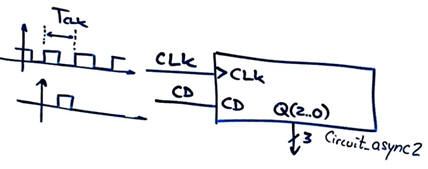Fig. 1. Symbol and schematic of the asynchronous circuit to be analysed. CLK is a rectangular wave of TCLK period. Clear direct (CD) is a single pulse any time the user like to initialise the circuit.Other similar analysis problems: As usual in CSD, before attempting this analysis project, we need to review materials, theory and other analogous examples of asynchronous and synchronous circuits.

- Use these tutorials to learn about specifications and how to use 1-bit memory cells: D_FF, JK_FF (and also RS_FF), T_FF.

- Circuit_Async is analysed in Lab5, Circuit_Async4,  Circuit_Sync1, Circuit_Async3

Other assignments (analysis problems A2.1, A2.2, ...)

2. Planning (up to three strategies to analyse the circuit)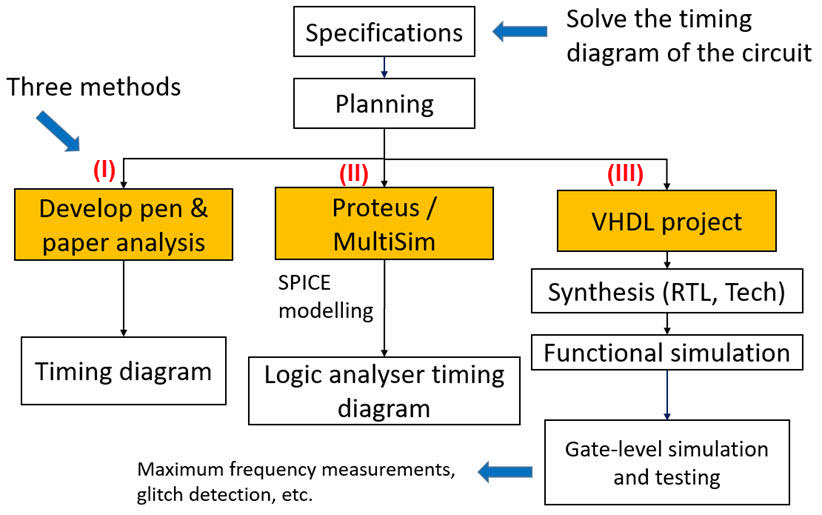Fig. 2. We get solutions determining how the circuit works comparing results form three methods.

3. Method 1 development

Pictures, notes, scanned materials, theory, etc. can be stored in this project location:

C:\CSD\P5\Circuit_async2\paper\(files)

General procedure and paper work for analysing circuits based on flip-flops.

a) Study how a single flip-flop works. Imagine values for control input T (or D, JK, RS) and try to obtain outputs applying function tables.

In this circuit there are T_FF and JK_FF flip-flops that work as explained in its tutorial.

b) Identify the number of CLK signals in the circuit. Is it asynchronous or synchronous? To draw the timing diagram, go step by step advancing in time by TCLK periods (circuit's time resolution). Apply an initial CD pulse to reset the system and start from a known state.

This is a video  that explains the problem planning and solutions, the last part of which shows how to deduce the timing diagram in paper.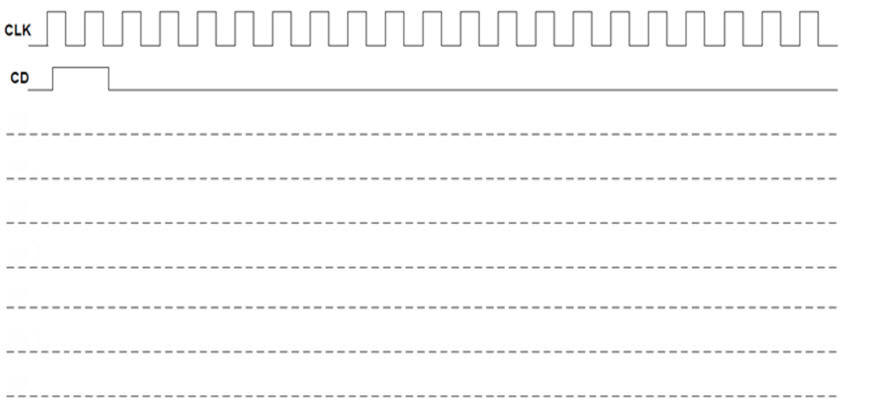Fig 3. Typical CLK and CD waveforms in a sheet of paper for most of Chapter 2 timing diagrams. Vertical dotted lines will be placed on CLK rising edges of interest.

This circuit contains two CLK signals. It is asynchronous, because rising edges are not going to happen exactly at the same time, thus complicating the analysis.

c) Be sure to identify what T (or D, JK, RS) input values are sampled at the CLK's rising edge. To do it, consider the small tCO (in the range of ns) associated to each output transition. Add text discussing what is happening after each CLK active.

K and T inputs are always sampled '1'. J sampled values will depend on Q evolution.

 NOTE: Explain using arrows and graphic annotations what happens and when and how does it work as time go advancing. Timing diagrams and solutions will not be considered valid without such explanations and discussions.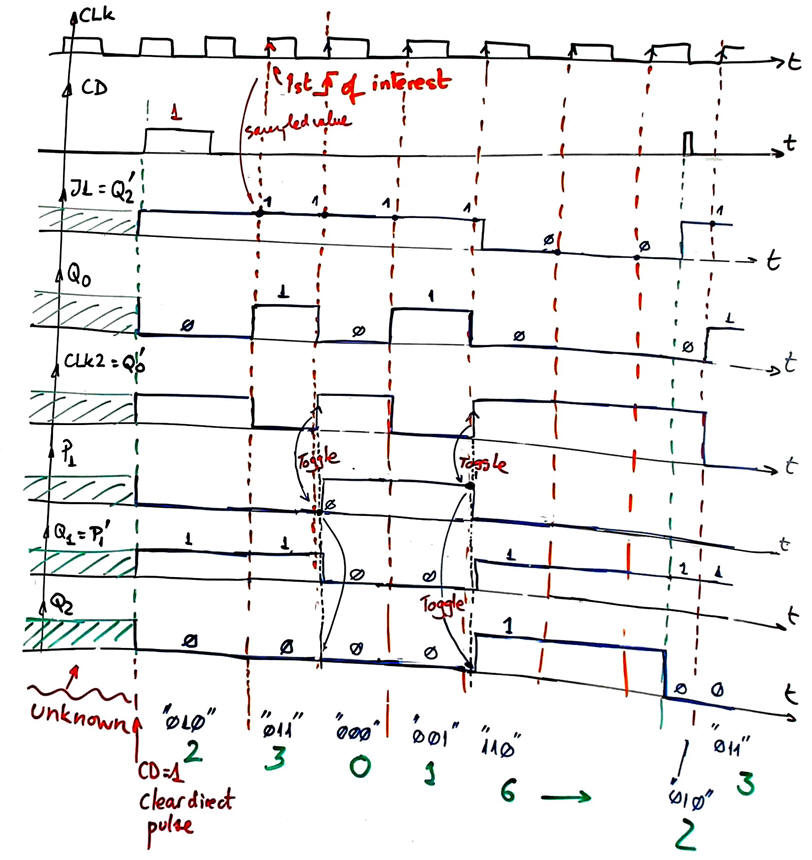Fig. 4. Example of timing diagram and analysis discussion.

d) Once the timing diagram is completed, determine the number of states and what is the binary output for each state.

This circuit is capable of memorising 5 states: 2 - 3 - 0 - 1 - 6

Discuss what may be the function or the circuit's application, if any. Is seems that it does not have a clear applications unless it is to generate a 5 number pattern at the CLK's speed.

What is the circuit's main problem, so that it must be rejected for precision applications? The problem is that gate delays has to be considered because of the two different CLK signals. In P6 we can design a canonical synchronous FSM to generate this pattern of numbers.

3. Method 2 development

Project location:

C:\CSD\P5\Circuit_async2\Proteus\(files)

Draw your Circuit_async.pdsprj and simulate in Proteus. Verify your solution using the logic analyser instrument. This a Proteus circuit to play with flop-flops as if we were in the laboratory building such circuits for real using classic 4000 CMOS series.

When picking parts from the library to mount your circuit, do this initialization --> Tool --> Global Annotator --> Total. Example adaptation Circuit_async2.pdsprj.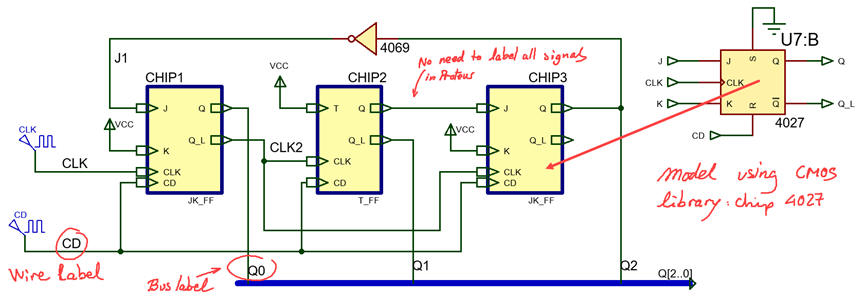Fig. 5. Example of circuit captured in Proteus.

You can discuss logic analyser results from screen captures or from printed versions as shown in Fig. 6.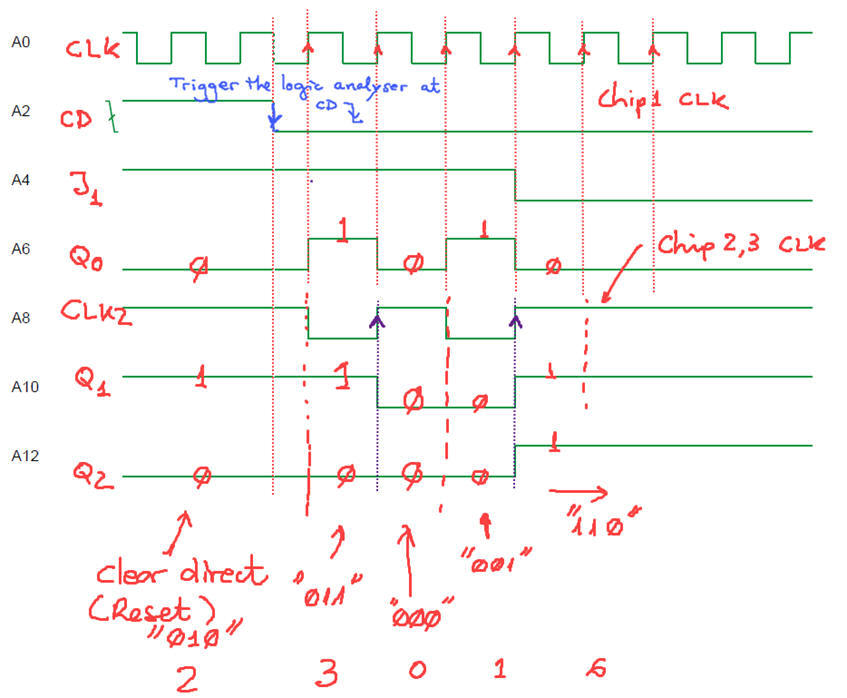Fig. 6. Example of circuit waveforms from the logic analyser printing.

Thus, this circuit does not generate periodic signals, but a single event sequence with numbers: "2 --> 3 --> 0 --> 1 --> 6" after CD pulse.

Measuring propagation delays in Proteus simulations is possible but time consuming and not practical, specially when we can do it much better using ModelSim gate-level simulations applying next analysis method 3.

3. Method 3 development

Project location:

C:\CSD\P5\Circuit_async2\VHDL\(files)

The internal architecture of the circuit proposed in Fig. 1 has to be fully annotated as in Fig. 7 before developing the VHDL project. This is a plan C2 project of three files.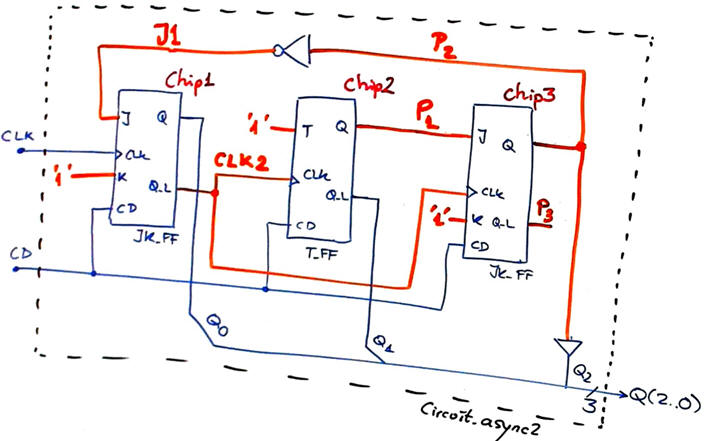Fig. 7. Circuit fully annotated and ready for translation to VHDL.

Translate the Fig 7 schematic to VHDL (Circuit_async.vhd). Toggle flip-flop description in VHDL is available in its tutorial T_FF. JK flip-flop description is available in  JK_FF.

Syntesise the project Circuit_Async2_prj for a MAXII EPM2210F324C3 chip (later on, you can change the target chip to a Cyclone IV EP4CE115F29C7 device and compare performances).

Inspect and analyse the RTL schematic generated by the synthesiser EDA tool as shown in Fig 8.Fig. 8. RTL produced by the synthesiser.

Inspect and analyse the technology schematic generated by the synthesiser EDA tool as shown in Fig 9. How many registers (D_FF) are used?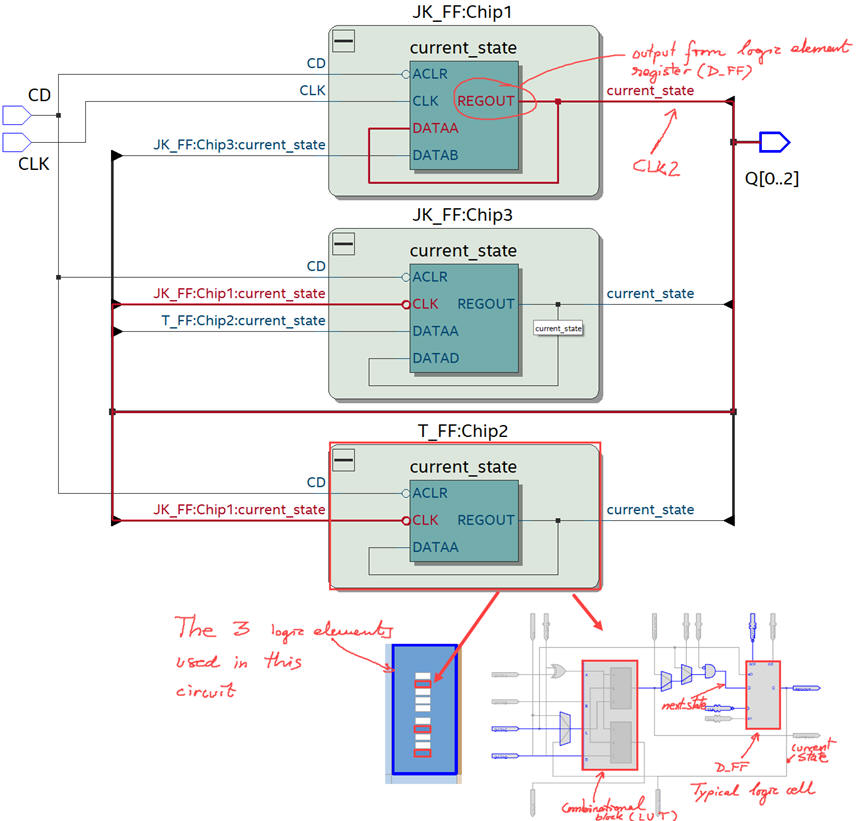Fig. 9 Technology schematic to be tested using gate-level simulations.

Fig. 10 shows the generalised VHDL testbench schematic that we have in mind to run simulations for our sequential systems under test. Remark the significant change replacing Chapter 1 Min_Pulse constant by this new Chapter 2 CLK_Period to define from now on time resolution. Any event shorter than a CLK period is not "seen" by the digital system.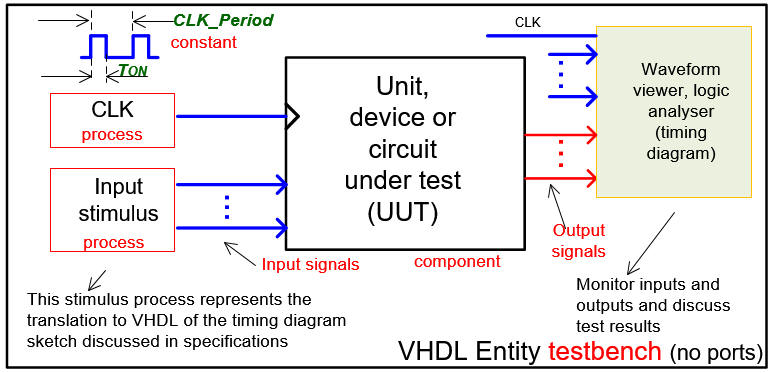Fig. 10. General testbench fixture for sequential systems, including at least two stimulus processes: one for the CLK and another for the remaining input ports.

Fig. 11 represents the inputs required in this experiment. A periodic CLK waveform of rectangular shape, for instance with a duty cycle = 25% and a clear direct CD pulse that can be repeated when necessary to initialise again the circuit. Make all the timing relative to the constant CLK_Period, that is the equivalent in Chapter 2 to the Chapter 1 contant Min_Pulse.Fig. 11. CLK and CD activity to be described in the testbench processes. From this activity the simulator will calculate outputs. Normal operation of the circuit can be inspected for example setting CLK_Period = 4 us. And detailed time measurements on signal transitions can be performed setting CLK_Period = 40 ns when testing the real technology view synthesised circuit for a given target chip (FPGA or CPLD).

Start the testbench template and add CLK and inputs activity translating input signals in the timing diagram. Thus, to test sequential systems at least two stimulus processes will be required: the CLK process and another process for all the other inputs. This is an example testbench file Circuit_async_tb.vhd.

Functional simulation. Run the EDA VHDL tool and demonstrate how the circuit works adding comments to the printed sheet of paper containing the waveforms.Fig. 12. Waveforms from functional simulation. This circuit looks like that is acting as a 4-bit binary counter in radix-2.

Gate-level simulation. Run the EDA VHDL tool using the same test bench and demonstrate CLK to output propagation delays (tCO) and calculate the maximum frequency of operation.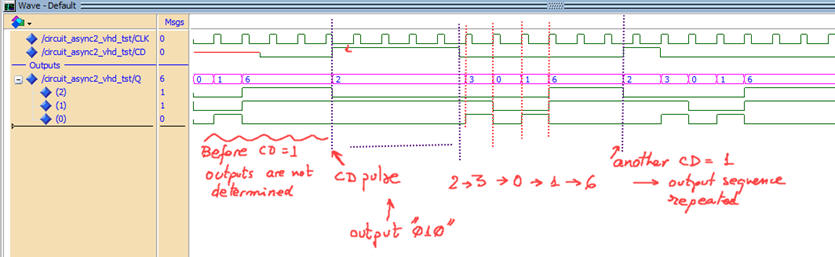Fig.13. Results from a gate-level simulation zooming all the test time to see that it works as expected.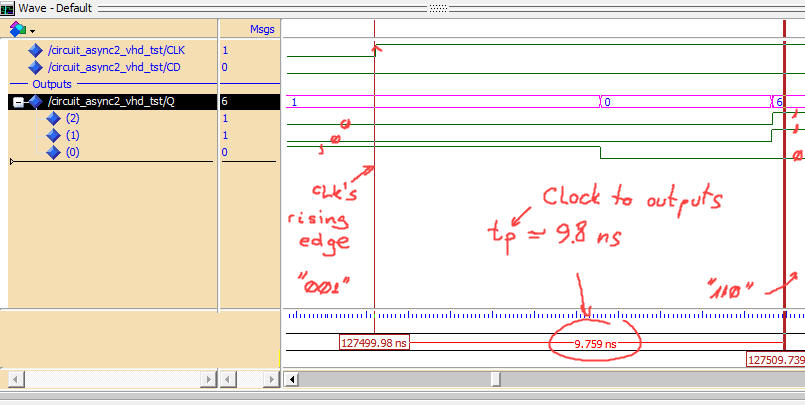Fig. 14. Results from a gate-level simulation focusing a single transition in ns time resolution window.

How about the timing analyser tool? Is is easy to use and obtain results in such circuits with several CLK signals?

4. Testing

We have demonstrated how the circuit works, comparing results from three analysis methods. Fig. 4, Fig. 6 and Fig. 12 are giving the same outputs.

5. Report

In this tutorial there are proposed three projects for comparing results

Method 1 requires sections 1 - 2 - 3 - 4.

Method 2 requires sections 1 - 2 - 3 - 4.

Method 3 requires sections 1 - 2 - 3 - 4 .

Theory and additional content may be included in section 1 on specifications of a given project.